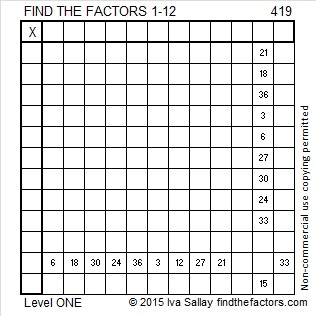# 419 and Level 1

2^419 (mod 419) = 2 so 419 is VERY LIKELY a prime number. Scroll down past the puzzle to know for sure.Print the puzzles or type the factors on this excel file: 12 Factors 2015-03-09

• 419 is a prime number.
• Prime factorization: 419 is prime.
• The exponent of prime number 419 is 1. Adding 1 to that exponent we get (1 + 1) = 2. Therefore 419 has exactly 2 factors.
• Factors of 419: 1, 419
• Factor pairs: 419 = 1 x 419
• 419 has no square factors that allow its square root to be simplified. √419 ≈ 20.4695How do we know that 419 is a prime number? If 419 were not a prime number, then it would be divisible by at least one prime number less than or equal to √419 ≈ 20.4695. Since 419 cannot be divided evenly by 2, 3, 5, 7, 11, 13, 17, or 19, we know that 419 is a prime number.